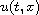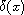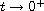Electron. J. Diff. Equ., Vol. 2015 (2015), No. 231, pp. 1-16.

### Similarity solutions of a replicator dynamics equation associated with a continuum of pure strategies Vassilis G. Papanicolaou, Kyriaki Vasilakopoulou

Abstract:
We introduce a nonlinear degenerate parabolic equation containing a nonlocal term. The equation serves as a replicator dynamics model where the set of strategies is a continuum. In our model the payoff operator (which is the continuous analog of the payoff matrix) is nonsymmetric and, also, evolves with time. We are interested in solutions u(t, x) of our equation which are positive and their integral (with respect to x) over the whole space is 1, for any t > 0. These solutions, being probability densities, can serve as time-evolving mixed strategies of a player. We show that for our model there is an one-parameter family of self-similar such solutions, all approaching the Dirac delta functionas.

Submitted August 24, 2015. Published September 10, 2015.
Math Subject Classifications: 35C06, 91A22, 91B52.
Key Words: Replicator dynamics model; nonlinear degenerate parabolic PDE; nonlocal term; probability densities evolving in time; self-similar solutions.

Show me the PDF file (265 KB), TEX file, and other files for this article.Vassilis G. Papanicolaou Department of Mathematics National Technical University of Athens Zografou Campus 157 80 Athens, Greece email: papanico@math.ntua.gr Kyriaki Vasilakopoulou Department of Mathematics National Technical University of Athens Zografou Campus 157 80 Athens, Greece email: kiriakivasilakopoulou@gmail.com